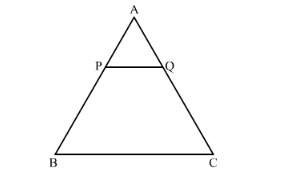# In the given figure, PQ || BC and AP : PB = 1 : 2. Find area ∆APQarea ∆ABC.

Question:

In the given figure, PQ || BC and AP : PB = 1 : 2. Find area ∆APQarea ∆ABC.Solution:

GIVEN: In the given figure PQ || BC, and AP: PB = 1:2

TO FIND: $\frac{\text { Area }(\mathrm{APQ})}{\text { Area }(\mathrm{ABC})}$

We know that according to basic proportionality theorem if a line is drawn parallel to one side of a triangle intersecting the other side, then it divides the two sides in the same ratio.

Since triangle APQ and ABC are similar

Hence, $\frac{\mathrm{AP}}{\mathrm{AB}}=\frac{\mathrm{AQ}}{\mathrm{AC}}=\frac{\mathrm{PQ}}{\mathrm{BC}}$

Now, it is given that $\frac{\mathrm{AP}}{\mathrm{PB}}=\frac{1}{2}$.

$\Rightarrow \mathrm{PB}=2 \mathrm{AP}$

So, $\frac{\mathrm{AP}}{\mathrm{AB}}=\frac{\mathrm{AP}}{\mathrm{AP}+\mathrm{PB}}=\frac{\mathrm{AP}}{\mathrm{AP}+2 \mathrm{AP}}=\frac{1}{3}$

Since the ratio of the areas of two similar triangle is equal to the ratio of the squares of their corresponding sides.

AreaAPQAreaABC=APAB2=132=19

$\frac{\text { Area }(\mathrm{APQ})}{\text { Area }(\mathrm{ABC})}=\frac{1}{9}$

Hence we got the result Area $(\triangle \mathrm{APB}):$ Area $(\triangle \mathrm{ABC})=1: 9$